# Python Pillow – ImageDraw模块

Python Pillow教程ImageDraw模块为Image Object提供简单的2D图形支持。一般来说，我们用这个模块来创建新的图像，注释或修饰现有的图像，并为网络使用而即时生成图形。

• 一个图像可以被很好地理解为一个二维的像素（图片元素）阵列。一个像素是被支持的最小的颜色点。
• ImageDraw使用的二维坐标系的原点在图像的左上角
• 我们使用的枕头颜色方案是RGB。RGB颜色的表示和支持是由模块ImageColor提供的。
• bitmap、OpenType或TrueType是可接受的用于文本注释的字体。
• 大多数绘图命令可能需要一个边界框参数，以指定该命令要应用的图像上的区域。
• 一个坐标序列可以表示为[ (x0, y0), (x1, y1), ... (xn, yn)]。
• 对于某些绘图命令，我们需要角度值。

## Python Pillow ImageDraw 例子

``````#Import required libraries
import sys
from PIL import Image, ImageDraw

#创建图像对象
im = Image.open("images/logo.jpg")

#画线
draw = ImageDraw.Draw(im)
draw.line((0, 0) + im.size, fill=128)
draw.line((0, im.size, im.size, 0), fill=128)

#显示图像
im.show()``````

## Python Pillow ImageDraw 输出## Python Pillow ImageDraw Canvas

• 一个ImageDraw是一个图像的Pillow可画表面（即画布）。
• ImageDraw.Draw(img)返回Image参数img的一个可画的画布表示。画布的背景是 "img "图像。

### Python Pillow ImageDraw Canvas 示例

``````#从Pillow包中导入所需的模块
from PIL import Image, ImageDraw, ImageFont

# 获得一个图像
base = Image.open('images/boy.jpg').convert('RGBA')

# 为文本制作一个空白图像，初始化为透明的文本颜色
txt = Image.new('RGBA', base.size, (255,255,255,0))

# 获得一种字体
fnt = ImageFont.truetype('E:/PythonPillow/Fonts/Pacifico.ttf', 40)

# 获得一个绘图环境
d = ImageDraw.Draw(txt)

# 绘制文本，一半不透明
d.text((14,14), "Tutorials", font=fnt, fill=（255,255,255,128）)

# 绘制文本，完全不透明
d.text((14,60), "Point", font=fnt, fill=(255,255,255))
out = Image.alpha_composite(base, txt)

#显示图像
out.show()``````

### Python Pillow ImageDraw Canvas 输出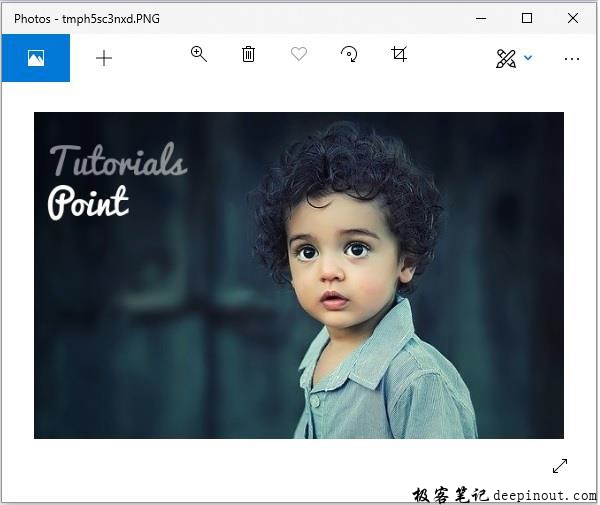## Python Pillow 使用ImageDraw模块绘制形状

ImageDraw模块允许我们创建不同的形状，首先用你要处理的图像创建一个绘图对象，然后应用它。我们可以使用'ImageDraw'模块绘制一些常见的形状，如下所示

### Python Pillow ImageDraw 画线

``draw.line(xy, fill=None, width=0)``

line()方法从边界盒xy和canvas的左上角到右下角画一条线。线条使用颜色填充。fill和width这两个参数的默认值分别为None和0，是可选的。

#### Python Pillow ImageDraw 画线 例子

``````from PIL import Image, ImageDraw

img = Image.new('RGB', (500, 300), (125, 125, 125))
draw = ImageDraw.Draw(img)
draw.line((200, 100, 300, 200), fill=(0, 0, 0), width=10)

img.show()``````

#### Python Pillow ImageDraw 画线 输出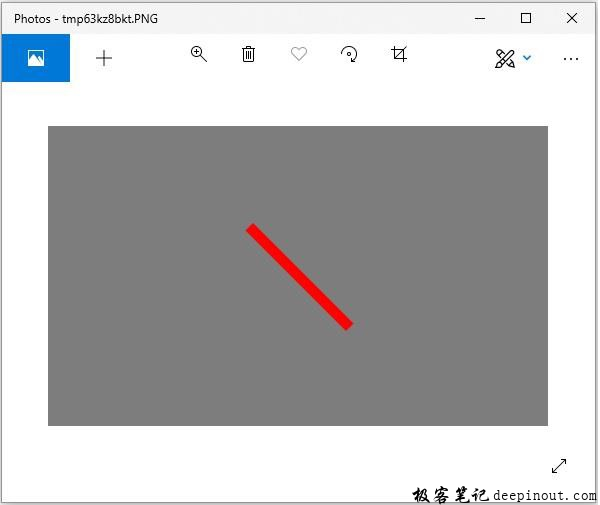### Python Pillow ImageDraw 画椭圆

``draw.ellipse(xy, fill=None, outline=None)``

ellipse()方法在绘制时画出由边界盒xy包围的椭圆。该形状使用颜色填充，周边使用颜色轮廓。默认值为None，参数fill和width是可选的。

#### Python Pillow ImageDraw 画椭圆 例子

``````from PIL import Image, ImageDraw

img = Image.new('RGB', (500, 300), (125, 125, 125))
draw = ImageDraw.Draw(img)

draw.ellipse((200, 125, 300, 200), fill=(255, 0, 0), outline=(0, 0, 0))
img.show()``````

#### Python Pillow ImageDraw 画椭圆 输出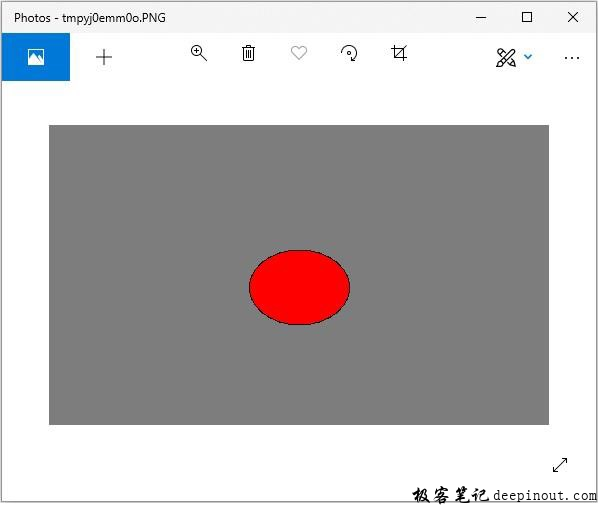### Python Pillow ImageDraw 画矩形

``draw.rectangle(xy, fill=None, outline=None)``

rectangle()方法在绘制时给定边界框xy，绘制矩形。该形状使用颜色填充，周长使用颜色轮廓。默认值为None，参数fill和width是可选的。

``````from PIL import Image, ImageDraw

img = Image.new('RGB', (500, 300), (125, 125, 125))
draw = ImageDraw.Draw(img)

draw.rectangle(
(200, 125, 300, 200),
fill=(255, 0, 0),
outline=(0, 0, 0))
img.show()``````

#### Python Pillow ImageDraw 画矩形 输出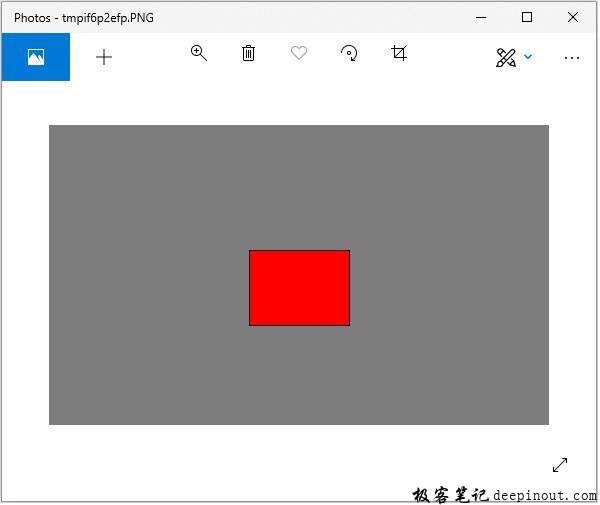### Python Pillow ImageDraw 画多边形

``draw.polygon(seq, fill=None, outline=None)``

polygon()方法绘制一个多边形，用直线将坐标序列的位置seq连接在一起。seq中的第一个和最后一个坐标也用一条直线连接。形状用颜色填充，周长用颜色勾勒。参数fill和outline是可选的，默认值为 None。

``````from PIL import Image, ImageDraw

img = Image.new('RGB', (500, 300), (125, 125, 125))
draw = ImageDraw.Draw(img)

draw.polygon(
((200, 200), (300, 100), (250, 50)),
fill=(255, 0, 0),
outline=(0, 0, 0))
img.show()``````

#### Python Pillow ImageDraw 画多边形 输出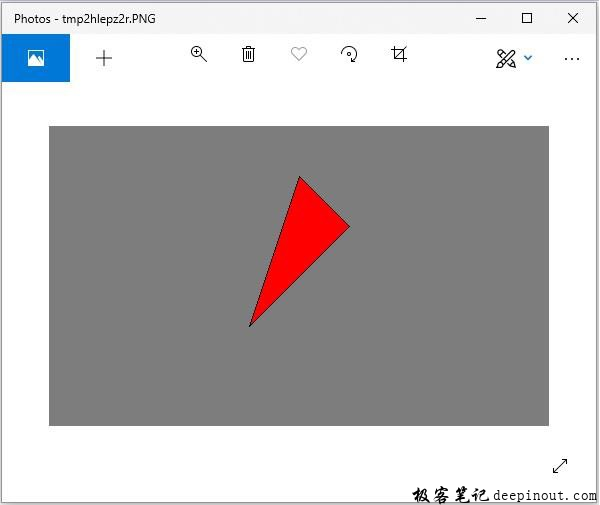• 微信订阅
• 回顶